# 一个接口快速测试小技巧(Python)

### 一个接口快速测试小技巧(Python)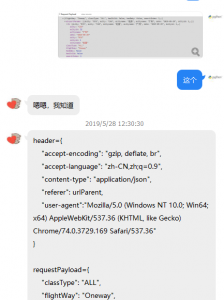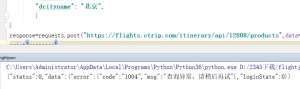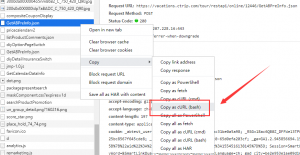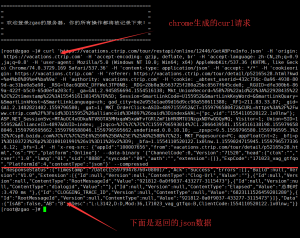–data-binary 以二进制的方式post数据
 –compressed 要求返回是压缩的形势 (using deflate or gzip)

```import re
import json
print('\t接口测试小工具 --curl转Python代码')
print('\t\t\tAuthor by: Zgao')
while True:
curl=input('请输入从Chrome中复制的curl(bash)：')
try:
info = curl.split('--data-binary')
url = re.search('[a-zA-z]+://[^\s]*', info).group()[:-1]
for i in info.split('-H')[1:]:
a = i.split(':', 1)
method = 'get'
except:
info = curl.split('--compressed')
url = re.search('[a-zA-z]+://[^\s]*', info).group()[:-1]
for i in info.split('-H')[1:]:
a = i.split(':', 1)
method = 'get'
data = {}

print('''
import requests

url = '{}'
data = {}

print(r.text)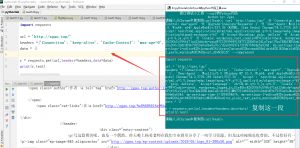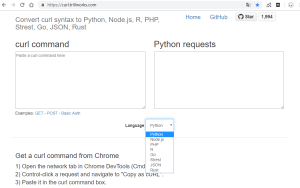微信赞赏支付宝赞赏